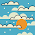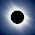# Difference between == and equals() method in Java - String Object

What is the difference between == and equals() method for comparing Objects in Java is one of the classical Interview Questions which appears now and then in many interviews? This question is mostly asked in conjunction with String because comparing String using == and equals() method returns different results. I have often seen along with other popular String  question e.g. StringBuffer vs StringBuilder, Why String is final etc.  Java is a pure object-oriented language and every object has one state and location in the memory and equals () and == are related to the state and location of the object, now in this article will try to understand this concept and difference between == and equals method in Java.

## What is equals method and == operator in Java

Both equals() method and the == operator is used to compare two objects in Java. == is an operator and equals() is method. But == operator compares reference or memory location of objects in the heap, whether they point to the same location or not.

Whenever we create an object using the operator new it will create new memory location for that object. So we use == operator to check memory location or address of two objects are same or not.

When we talk about equals() method the main purpose is to compare the state of two objects or contents of the object. But there is one relation between this two is default implementation of equals() method work like == means it will check the memory reference of the object if they point to the same location then two objects are equals and it is defined in Object class.

As we know java.lang.Object class is parent for every other object so default  implementation is common for every object but if we want to override the method and want to give own implementation  for checking the equality of two objects we can do, and most of the Java  classes have their own implementation for equals method where they check the contents of the object .

Btw, if you are not familiar with essential OOP concepts like overloading and overriding then you should first check The Complete Java Masterclass to understand. That will help you to understand the difference between the difference between equals() method in Object class and String class.

For example  java.lang.String class overrides the equals() and hashcode method and in the overridden method, it will check that two string contains same value or character if yes then they are equals otherwise not equal.

## Difference between == and equals method in Java

Now we know what equals method, how it works and What is equality operator (==) and How it compare objects, it's time to compare them. Here is some worth noting difference between equals() method and == operator in Java:

·          First difference between them is, equals() is a method defined inside the java.lang.Object class and == is one type of operator and you can compare both primitive and objects using equality operator in Java.

·          Second difference between equals and == operator is that, == is used to check reference or memory address of the objects whether they point to the same location or not, and equals() method is used to compare the contents of the object e.g. in case of comparing String its characters, in case of Integer it's their numeric values etc.

You can define your own equals method for domain object as per business rules e.g. two Employee objects are equal if there EmployeeId is same.

·          Third difference between equals and the == operator is that You can not change the behavior of == operator but we can override equals() method and define the criteria for the objects equality.

Let clear all these differences between equals and == operator using one Java example :

String s1=new String("hello");
String s2=new String("hello");

Here we have created two string s1 and s2 now will use == and equals () method to compare these two String to check whether they are equal or not.

First, we use equality operator  == for comparison which only returns true if both reference variables are pointing to the same object.

if(s1==s2) {
System.out.printlln("s1==s2 is TRUE");
} else{
System.out.println("s1==s2 is FALSE");
}

The output of this comparison is FALSE because we have created two objects which have a different location in the heap so == compare their reference or address location and return false. Now if we use equals method to check their equivalence what will be the output

if(s1.equals(s2)) {
System.out.println("s1.equals(s2) is TRUE");
} else {
System.out.println("s1.equals(s2) is FALSE");
}

The output of this comparison is TRUE because of java.lang.String class has already overridden the equals() method of Object class and check that contents are same or not because both have same value hello so they are equal according to String class equals() method.

A picture is worth of thousands of words and here is a picture which explains whatever I have said in the above paragraph about equals() vs == operator in case of String in Java:

### Point to remember:If you have not overridden equals() method in a user-defined object,  it will only compare the reference or memory address as defined in default equals() method of java.lang.Object class and return true only if both reference variable points to the same object.

So in a user-defined class, both equals() and == operator behave similarly but that may not be logically correct and that’s why we should always define the equivalence criteria for custom or domain objects.

That’s all on the difference between equals() method and == operator in Java. Both can compare objects for equality but equals() is used for logical and business logic comparison while == mostly for object reference comparison in Java.

Further Learning
The Complete Java Masterclass
Java Fundamentals: The Java Language
Java Interview Guide: 200+ Interview Questions and Answers

Other Java articles and Interview questions from Java 67
Hashtable vs HashMap in Java

Thanks for reading this article so far. If you like this core Java interview question and my explanation then please share with your friends and colleagues. If you have any doubt or feedback then please drop a note.

1.I think most important difference between equals and == operator in Java is that former is method while other is operator, isn't it ?

2.== operator returns true or false why

3.As per your article "diff b/w string literal and string object", you sed that when you use new operator it creates a separate new object, so then in the above first snipets, how come the object of String are equal w.r.t reference ,

1.4.